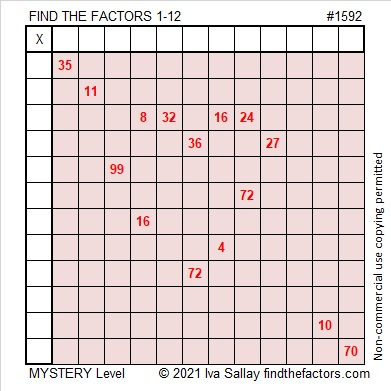# 1592 One More Valentine

### Today’s Puzzle:

I made this mystery level puzzle into one more valentine. Love can seem tricky sometimes, but I hope you enjoy working on it.### Factors of 1592:

• 1592 is a composite number.
• Prime factorization: 1592 = 2 × 2 × 2 × 199, which can be written 1592 = 2³ × 199.
• 1592 has at least one exponent greater than 1 in its prime factorization so √1592 can be simplified. Taking the factor pair from the factor pair table below with the largest square number factor, we get √1592 = (√4)(√398) = 2√398.
• The exponents in the prime factorization are 3 and 1. Adding one to each exponent and multiplying we get (3 + 1)(1 + 1) = 4 × 2 = 8. Therefore 1592 has exactly 8 factors.
• The factors of 1592 are outlined with their factor pairs in the graphic below.### More about the Number 1592:

1599 is the difference of two squares two different ways:
399² – 397² = 1592, and
201² – 197² = 1592.